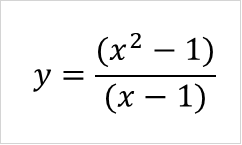ChaptersA Symbol for the limit B As the inputs, x, approach a specific value a C The function we want to find the limit for

The notation for approaching the value a on the right and left can be found below.A B Approach from right side Approach from left side

## Approach from the Left

Sometimes, the function at the specified value a doesn’t exist. Take a look at some examples below.

 Function Results is UnknownWhen this happens, we can instead get as close to the specific value a as possible. Take a look.x y 0.8 1.8000 0.9 1.9000 0.99 1.9900 0.999 1.9990 0.9999 1.9999

As we approach 1, we see that our value gets closer and closer to 2. We can say that our limit is 2.

## Approach from the Right

In the previous section, we wanted to approach 1. So, we plugged in values that were closer and closer to 1. In that example, we approached 1 from the left side. Take a look at why:We can also approach our a value from the right side. Take a look at the result.

 x y 1.1 2.1000 1.01 2.0100 1.001 2.0010 1.0001 2.0001

As you can see, we get the same result: as we approach 1 form the right side, we get closer and closer to two.

## Indeterminate Forms

Indeterminate forms have unknown values. This means we do know their true value, whether they are undefined or simply unknown. We can typically divide common indeterminate forms into three groups.A B C Fraction Standard Power

## Problem 1

In this problem, we will review what you have learned about indeterminate forms. Given the following table, label each limit that will result an indeterminate form as a approaches 0.

 123## Problem 2

Take a look at the following limit problem.Taking what you’ve learned about approaching limits, approach the value a from the right. Next, approach the value a from the left.

## Problem 3

Taking what you know about finding the limit, lets try to find the limit of a function as it approaches infinity.Keep in mind that for this problem you might have to approach the limit form the right or left, depending on what result you get.

## Problem 4

In this problem, we will put together some more knowledge on indeterminate forms. Take the following function.Find the limit of the function and then graph the function as it approaches its limit.

## Solution Problem 1

In this problem, you were asked to review what you learned about indeterminate forms. Given table, you had to label each limit that will result an indeterminate form as a approaches 0.1Indeterminate form 2Indeterminate form 3Regular

## Solution Problem 2

In this problem, you were asked to look at the limit problem and approach the value a from the right and from the left.

First, we plug in values that approach 2 from the left.

 x y 1 6 1.5 4.666667 1.9 4.105263 1.99 4.01005 1.999 4.001001

Here, we can see the y value gets closer and closer to 4.Let’s approach the value 2 from the right now and see if the same pattern occurs.

 x y 2.001 3.999 2.01 3.990 2 4.000 2.1 3.905 2.5 3.600

Here, we’re also getting closer to 4.We can see the approach from the right side.

## Solution Problem 3

In this problem, you were asked to take what you learned about finding the limit and use it to try to find the limit of a function as it approaches infinity. In this problem, we didn’t specify whether you had to approach the limit form the right or left.

Let’s first plug infinity into the problem.Here, we encounter a problem: infinity is endless and therefore unknown/undefined. However, we can approximate infinity by plugging in higher and higher values into thisterm.

 x y 10 0.1 100 0.01 1000 0.001 90000 1.11E-05

As you can see, when we approach higher and higher values of x, we get closer and closer to zero.Because this term is approximately zero, we can say that the limit is approximately 2.## Solution Problem 4

In this problem, we were asked to find the limit of the function and then graph the function as it approaches its limit. We can already see that plugging in this a value will result in an indeterminate form.However, we can try plugging in values that approach zero into the x place.

 x y 1 1 0.5 2 0.01 100 0.001 1000 0.0001 10000

We can see that as we plug in smaller and smaller values, the y value approaches bigger and bigger numbers. We can also see this visually:Without using l’Hopital’s rule for finding the limit of indeterminate forms, we can say that as x approaches zero, the limit approaches infinity.The platform that connects tutors and students4.00 (2 rating(s))Loading...